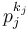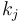# Nilpotency-forcing number

This article defines a property that can be evaluated for natural numbers

## Contents

BEWARE! This term is nonstandard and is being used locally within the wiki. [SHOW MORE]

## Definition

A natural number$n$ is said to be nilpotency-forcing or nilpotence-forcing if the following equivalent conditions hold:

1. Every group of order$n$ is nilpotent
2. Every group of order$n$ is a direct product of its Sylow subgroups
3. Every prime divisor of$n$ is Sylow-direct
4. Every prime divisor of$n$ is Sylow-unique
5. Suppose$p_i, p_j$ are prime divisors of$n$ and$p_j^{k_j}$ is the largest power of$p_j$ dividing$n$. Then, the order of$p_j$ modulo$p_i$ exceeds$k_j$. In other words,$p_i$ does not divide$p_j^l - 1$ for$1 \le l \le k_j$.

For proof of the equivalence of definitions, see classification of nilpotency-forcing numbers.